Simpact Cyan Population based event driven simulation using mNRM
EventBase Class Reference

This is the base class for events in the mNRM algorithm. More...

#include <eventbase.h>

Inheritance diagram for EventBase: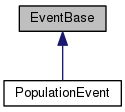[legend]

## Public Member Functions

virtual void fire (State *pState, double t)
This function will be called when the event fires, so this should most likely be re-implemented in your own event. More...

bool willBeRemoved () const
Check if the event has been marked for deletion, can avoid a call to the random number generator to create a new random number. More...

void setWillBeRemoved (bool f)
When an event has fired, by default a new internal fire time will be calculated; setting this flag avoids this which can be useful if the event isn't going to be used again. More...

## Protected Member Functions

virtual double getNewInternalTimeDifference (GslRandomNumberGenerator *pRndGen, const State *pState)
This function will be called to generate a new internal time difference. More...

virtual double calculateInternalTimeInterval (const State *pState, double t0, double dt)
This function should map the real world time interval onto an internal time interval and return it. More...

virtual double solveForRealTimeInterval (const State *pState, double Tdiff, double t0)
This function should calculate the real world time interval that corresponds to the value of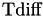. More...

## Detailed Description

This is the base class for events in the mNRM algorithm.

To create an actual event implementation, these functions should be reimplemented:

• EventBase::fire: this function is executed when the event fires
• EventBase::getNewInternalTimeDifference: this generates a random internal time interval, corresponding to the initial value of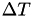for this event. If not re-implemented, a number from the exponential distribution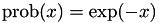is used for this.
• EventBase::calculateInternalTimeInterval: this should calculate the internal time intervalaccording to the equation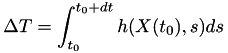where the state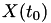is specified as the parameter pState. The values for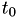and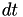are passed as parameters to this function as well. If this function is not re-implemented a very straightforward mapping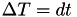is used.
• EventBase::solveForRealTimeInterval: for the specified value of(is the parameter Tdiff), this function must calculate and return the value ofso that the following integral equation is fulfilled:(the equation is the same as above, but now it solved for. Again, the stateis specified as the parameter pState andis a parameter to this function as well. If not re-implemented, this function uses the very straightforward mapping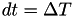.

As discussed on the main page, negative real-world times are used to signal that a recalculation is necessary, so in a simulation events can only fire at positive times.

Since this class by default uses an exponential distribution, the implementation of your own event will probably only need the calculateInternalTimeInterval and solveForRealTimeInterval to implement the specific hazard to use for the event. Of course, most likely the fire function will need to be implemented as well.

If you want to schedule events using a real world time directly, you can just implement the getNewInternalTimeDifference function to generate a real world time interval until the real world event fire time. Because the calculateInternalTimeInterval and solveForRealTimeInterval function by default use the trivialmapping onto real world times, you won't need to implement them to make the event fire at the correct time.

## Member Function Documentation

 double EventBase::calculateInternalTimeInterval ( const State * pState, double t0, double dt )
protectedvirtual

This function should map the real world time interval onto an internal time interval and return it.

Basically the function should calculate the integralwhereandare specified as parameters andis the state of the simulation specified by pState.

If not re-implemented, this function just returns the value of, corresponding to the trivial mapping.

 void EventBase::fire ( State * pState, double t )
virtual

This function will be called when the event fires, so this should most likely be re-implemented in your own event.

Parameters
 pState The current simulation state when the event fires. The firing of the event can change this state. t The time at which the event fires, so this is the current simulation time (a real world time).
 double EventBase::getNewInternalTimeDifference ( GslRandomNumberGenerator * pRndGen, const State * pState )
protectedvirtual

This function will be called to generate a new internal time difference.

By default, as is common in the mNRM, a random number from an exponential distributionis used for this, but by re-implementing this function you can change this.

For example, if you just want to make an event fire at a specific real world time, this function should just return the interval until that time (the current simulation time can be obtained from the State::getTime function). In that case you don't even need to re-implement EventBase::calculateInternalTimeInterval and EventBase::solveForRealTimeInterval since their default implementation is the trivialmapping between internal time and real world time.

 void EventBase::setWillBeRemoved ( bool f )
inline

When an event has fired, by default a new internal fire time will be calculated; setting this flag avoids this which can be useful if the event isn't going to be used again.

 double EventBase::solveForRealTimeInterval ( const State * pState, double Tdiff, double t0 )
protectedvirtual

This function should calculate the real world time interval that corresponds to the value of.

The function should returnthat solves the equation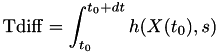whereandare specified as parameters andis the state of the simulation specified by pState.

If not re-implemented, this function just returns the value of, corresponding to the trivial mapping.

 bool EventBase::willBeRemoved ( ) const
inline

Check if the event has been marked for deletion, can avoid a call to the random number generator to create a new random number.

The documentation for this class was generated from the following files: